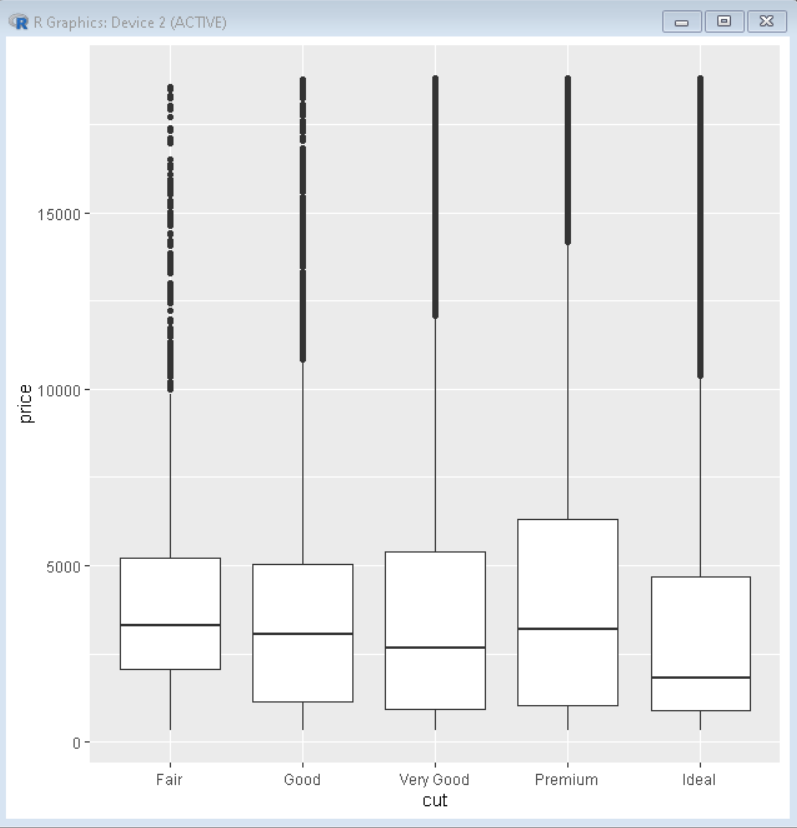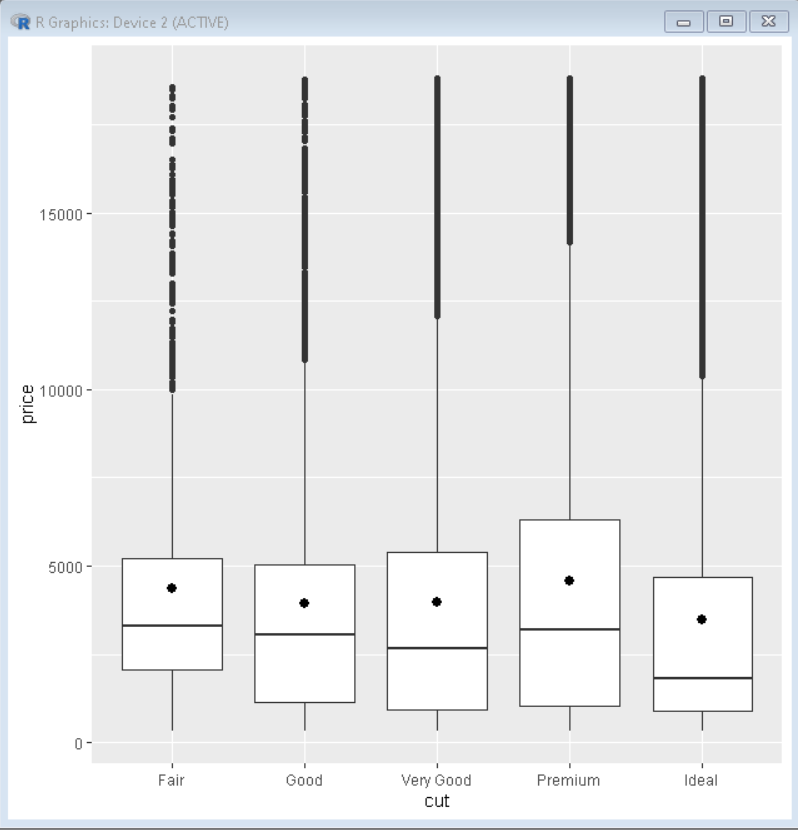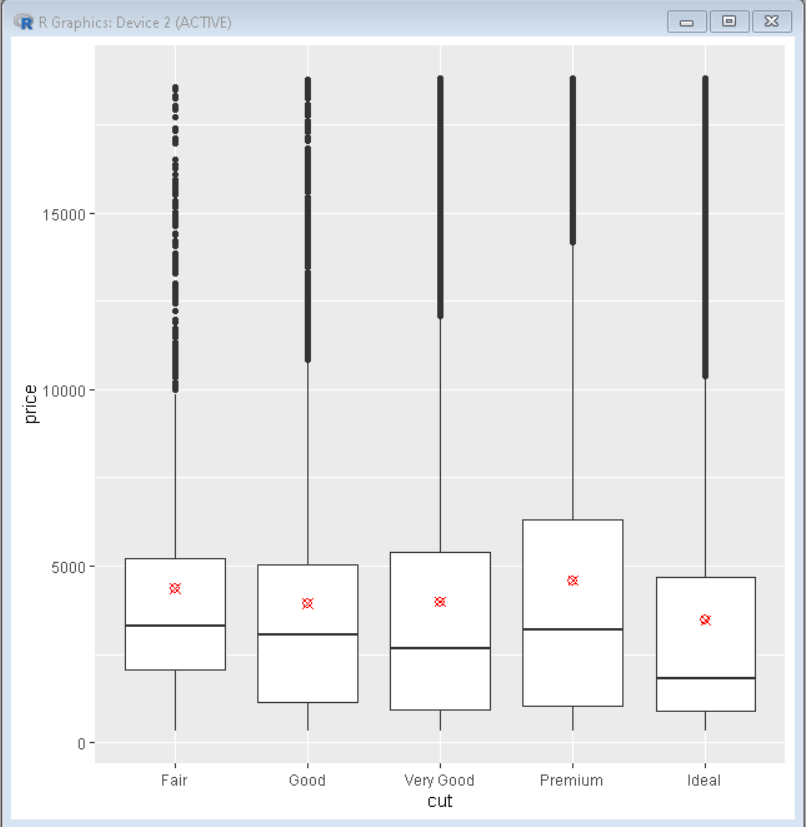# How To Show Mean Value in Boxplots with ggplot2?

In this article, we will discuss how to show mean value in Boxplot with ggplot2 using R programming language.

Firstly, we will create a basic boxplot using the geom_boxplot() function of the ggplot2 package and then do the needful, so that the difference is apparent.

Syntax:

ggplot() + geom_boxplot()

Example: Basic boxplot

## R

 `# load library tidyverse ` `library``(tidyverse) ` `library``(ggplot2) ` ` `  `# basic boxplot ` `ggplot``(diamonds, ``aes``(x=cut, y=price)) + ` ` `  `# geom_boxplot is used to plot the boxplot ` `  ``geom_boxplot``()`

Output:In order to show mean values in boxplot using ggplot2, we use the stat_summary() function to compute new summary statistics and add them to the plot. We use stat_summary() function with ggplot() function.

Syntax:

stat_summary(mapping = NULL, data = NULL, geom = “pointrange”, position = “identity”, color=”value”, shape=”value”,…)

Example: Adding mean value to boxplot

## R

 `# load libraries ` `library``(ggplot2) ` `library``(tidyverse) ` ` `  `# basic boxplot ` `ggplot``(diamonds, ``aes``(x=cut, y=price)) + ` ` `  `# geom_boxplot is used to plot the boxplot ` `  ``geom_boxplot``() + ` ` `  `# stat_summary computes the statistics summary ` `# fun.y arguments as mean determines that  ` `# statistical summary will be mean of y-axis ` `stat_summary``(fun.y=``"mean"``)`

Output:In the output produced above, the point in the center of the boxplot shows the variation of the mean of the y-axis for each category of data on the x-axis.

We can also change the color and shape of the mean mark using the color and shape parameter of the stat_summary() function. This helps us visualize the data better by distinguishing the mark from other symbols.

## R

 `# load library tidyverse ` `library``(tidyverse) ` `library``(ggplot2) ` ` `  `# basic boxplot ` `ggplot``(diamonds, ``aes``(x=cut, y=price)) + ` ` `  `# geom_boxplot is used to plot the boxplot ` `  ``geom_boxplot``() + ` ` `  `# stat_summary computes the statistics summary ` `stat_summary``(fun.y=``"mean"``,color=``"red"``, shape=13)`

Output:Whether you're preparing for your first job interview or aiming to upskill in this ever-evolving tech landscape, GeeksforGeeks Courses are your key to success. We provide top-quality content at affordable prices, all geared towards accelerating your growth in a time-bound manner. Join the millions we've already empowered, and we're here to do the same for you. Don't miss out - check it out now!

Previous
Next